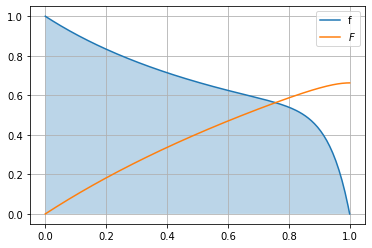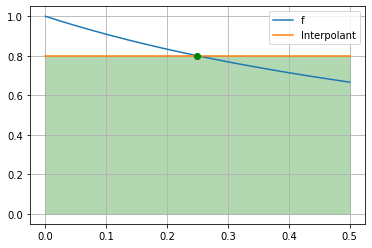# Accuracy of Newton-Cotes¶

In :
import numpy as np
import numpy.linalg as la
import matplotlib.pyplot as pt


A function to make Vandermonde matrices:

(Note that the ordering of this matrix matches the convention in our class but disagrees with np.vander.)

In :
def vander(nodes, ncolumns=None):
if ncolumns is None:
ncolumns = len(nodes)
result = np.empty((len(nodes), ncolumns))
for i in range(ncolumns):
result[:, i] = nodes**i
return result


Fix a set of nodes:

In :
 nodes = [0.5] # Midpoint
# nodes = 
#nodes = [0, 1] # Trapezoidal
#nodes = [0, 0.5, 1] # Simpson's
#nodes = [0, 1/3, 1]


Find the weights for the Newton-Cotes rule for the given nodes on $[0,1]$:

In :
(a, b) = (0, 1)
nodes = np.array(nodes)
n = len(nodes)

degs = np.arange(n)
rhs = 1/(degs+1)*(b**(degs+1 - a**(degs+1)))
weights = la.solve(vander(nodes).T, rhs)
print(weights)

[1.]


Here is a function and its definite integral from $0$ to $x$:

$$F(x)=\int_0^x f(\xi)d\xi$$

In :
fdeg = 15
def f(x):
return sum((-x)**i for i in range(fdeg + 1))

def F(x):
return sum(
(-1)**i*1/(i+1)*(
(x)**(i+1)-0**(i+1)
)
for i in range(fdeg + 1))


Plotted:

In :
plot_x = np.linspace(0, 1, 200)

pt.plot(plot_x, f(plot_x), label="f")
pt.fill_between(plot_x, 0*plot_x, f(plot_x),alpha=0.3)
pt.plot(plot_x, F(plot_x), label="$F$")
pt.grid()
pt.legend(loc="best")

Out:
<matplotlib.legend.Legend at 0x11c82f6d0>This here plots the function, the interpolant, and the area under the interpolant:

In :
# fix nodes
h = 0.5
x = nodes * h

# find interpolant
coeffs = la.solve(vander(x), f(x))

# evaluate interpolant
plot_x = np.linspace(0, h, 200)
interpolant = vander(plot_x, len(coeffs)) @ coeffs

# plot
pt.plot(plot_x, f(plot_x), label="f")
pt.plot(plot_x, interpolant, label="Interpolant")
pt.fill_between(plot_x, 0*plot_x, interpolant, alpha=0.3, color="green")
pt.plot(x, f(x), "og")
pt.grid()
pt.legend(loc="best")

Out:
<matplotlib.legend.Legend at 0x11c91df10>Compute the following:

• The true integral as true_val (from F)
• The quadrature result as quad (using x and weights and h)
• The error as err (the difference of the two)

(Do not be tempted to compute a relative error--that has one order lower.)

Compare the error for $h=1,0.5,0.25$. What order of accuracy do you observe?

In :
errors = []

for h in [1, 0.5, 0.25, 0.125, 0.125*0.5, 1/32]:
true_val = F(h)
quad = h * weights @ f(h * nodes)

errors.append(error)

1 0.6628718503718505 0.666656494140625 0.0037846437687745205
0.5 0.405464803170835 0.39999999990686774 0.005464803263967255
0.25 0.22314355131143945 0.22222222222222143 0.0009213290892180204
0.125 0.11778303565638346 0.11764705882352941 0.0001359768328540456
0.0625 0.06062462181643485 0.06060606060606061 1.8561210374239134e-05
0.03125 0.030771658666753687 0.03076923076923077 2.427897522916389e-06


Estimate the order of accuracy:

We assume that the error depends on the mesh spacings $h$ as $E(h)\approx C h^p$ for some unknown power $p$. Taking the $\log$ of this approximate equality reveals a linear function in $p$: $$E(h) \approx C h^p \quad \iff \quad \log E(h) \approx \log(C) + p\log(h).$$ You can now either do a least-squares fit for $\log C$ and $p$ from a few data points $(h,E(h))$ (more accurate, more robust), or you can use just two grid sizes $h_1$ and $h_2$, and estimate the slope: (less accurate, less robust) $$p \approx \frac{ \log(\frac{E(h_2)}{E(h_1)}) } {\log(\frac{h_2}{h_1})}.$$ This is called the empirical order of convergence or EOC.

In :
for i in range(len(errors)-1):
print(np.log(errors[i+1]/errors[i])/np.log(1/2))

1.9068441979782607
2.5605266307873995
2.7603285008344334
2.8729980764064282
2.9345113566351206